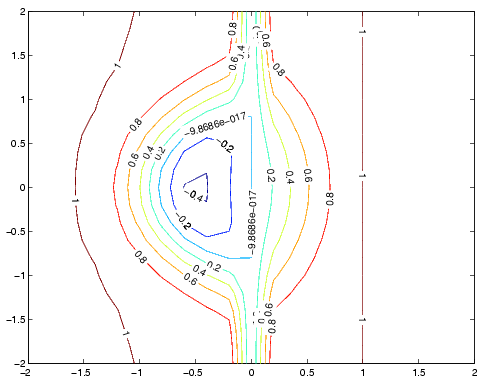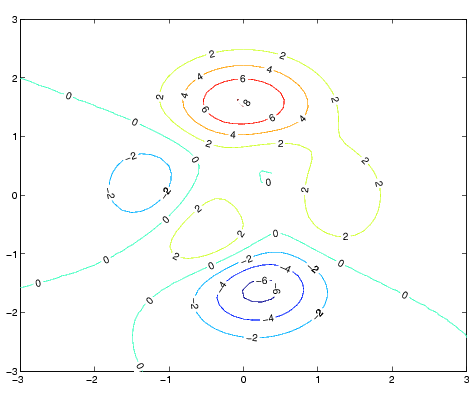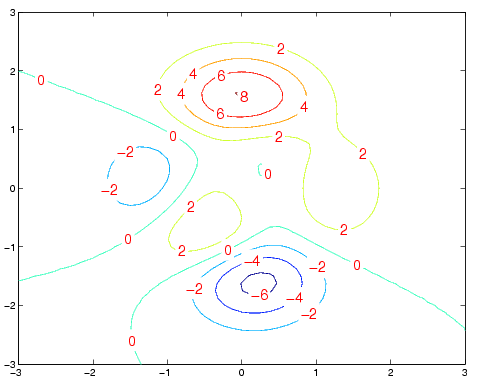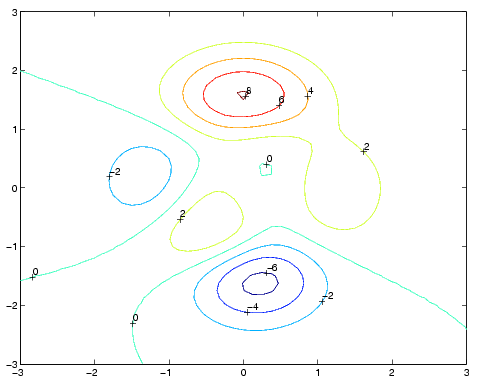MATLAB Function Referenceclabel

Contour plot elevation labels

Syntax

• ```clabel(C,h)
clabel(C,h,v)
clabel(C,h,'manual')

clabel(C)
clabel(C,v)
clabel(C,'manual')

text_handles = clabel(...)
clabel(...,'`PropertyName`',propertyvalue,...)
clabel(...'LabelSpacing',`points`)
```

Description

The `clabel` function adds height labels to a two-dimensional contour plot.

```clabel(C,h) ``` rotates the labels and inserts them in the contour lines. The function inserts only those labels that fit within the contour, depending on the size of the contour.

```clabel(C,h,v) ``` creates labels only for those contour levels given in vector `v`, then rotates the labels and inserts them in the contour lines.

```clabel(C,h,'manual') ``` places contour labels at locations you select with a mouse. Press the left mouse button (the mouse button on a single-button mouse) or the space bar to label a contour at the closest location beneath the center of the cursor. Press the Return key while the cursor is within the figure window to terminate labeling. The labels are rotated and inserted in the contour lines.

```clabel(C) ``` adds labels to the current contour plot using the contour array `C` output from `contour`. The function labels all contours displayed and randomly selects label positions.

```clabel(C,v) ``` labels only those contour levels given in vector `v`.

```clabel(C,'manual') ``` places contour labels at locations you select with a mouse.

```text_handles = clabel(...) ``` returns the handles of text objects created by clabel. The `UserData` properties of the text objects contain the contour values displayed. If you call `clabel` without the `h` argument, text_handles also contains the handles of line objects used to create the `'+'` symbols.

```clabel(...,'PropertyName',propertyvalue,...) ``` enables you to specify text object property/value pairs for the label strings. (See text properties.)

```clabel(...'LabelSpacing',points) ``` specifies the spacing between labels on the same contour line, in units of points (72 points equal one inch).

Remarks

When the syntax includes the argument `h`, this function rotates the labels and inserts them in the contour lines (see Examples). Otherwise, the labels are displayed upright and a `'+' `indicates which contour line the label is annotating.

Examples

Generate, draw, and label a simple contour plot.

• ```[x,y] = meshgrid(-2:.2:2);
z = x.^exp(-x.^2-y.^2);
[C,h] = contour(x,y,z);
clabel(C,h);```

Label a contour plot with label spacing set to 72 points (one inch).

• ```[x,y,z] = peaks;
[C,h] = contour(x,y,z);
clabel(C,h,'LabelSpacing',72)```

Label a contour plot with 15 point red text.

• ```[x,y,z] = peaks;
[C,h] = contour(x,y,z);
clabel(C,h,'FontSize',15,'Color','r','Rotation',0)```

Label a contour plot with upright text and `'+' `symbols indicating which contour line each label annotates.

• ```[x,y,z] = peaks;
C = contour(x,y,z);
clabel(C)```

See Also

`contour`, `contourc`, `contourf`

Annotating Plots for related functions

Drawing Text in a Box for an example that illustrates the use of contour labels

© 1994-2005 The MathWorks, Inc.